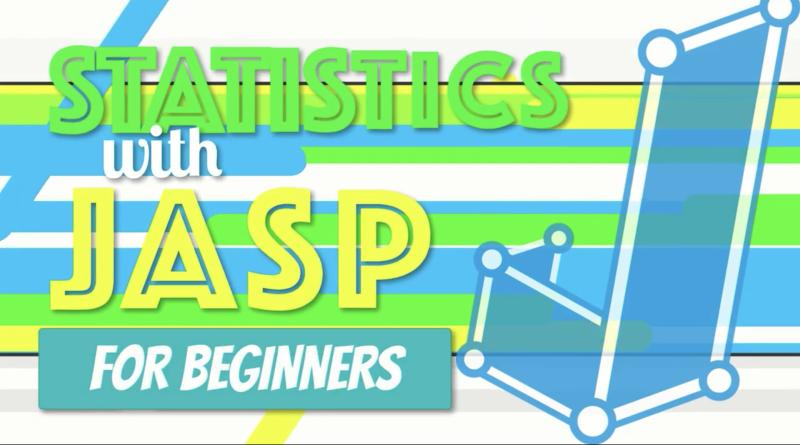# Video: How to Do Simple Linear Regression in JAS

Video (23:18) from Research by Design in which, “Exploring our data about burnout and job satisfaction, we predict an outcome with a single variable using simple linear regression in JASP. I explain how regression works, then open an SPSS dataset in JASP. We explore the assumptions of homoscedasticity and linearity with a scatterplot and normality using a Shapiro-Wilk test. Later we examine the residual statistics for outliers and use the Durbin-Watson test to check for independence of observations. Assumptions met, we conduct the simple linear regression in JASP, interpret the results, and write up the findings in APA style. I also answer the question: “What is the difference between capital R and lower case r, for reporting?”

If you’d like to have any of the files used in the video, you can access them here.

You can find other videos from the Statistics with JASP for Beginners series here.